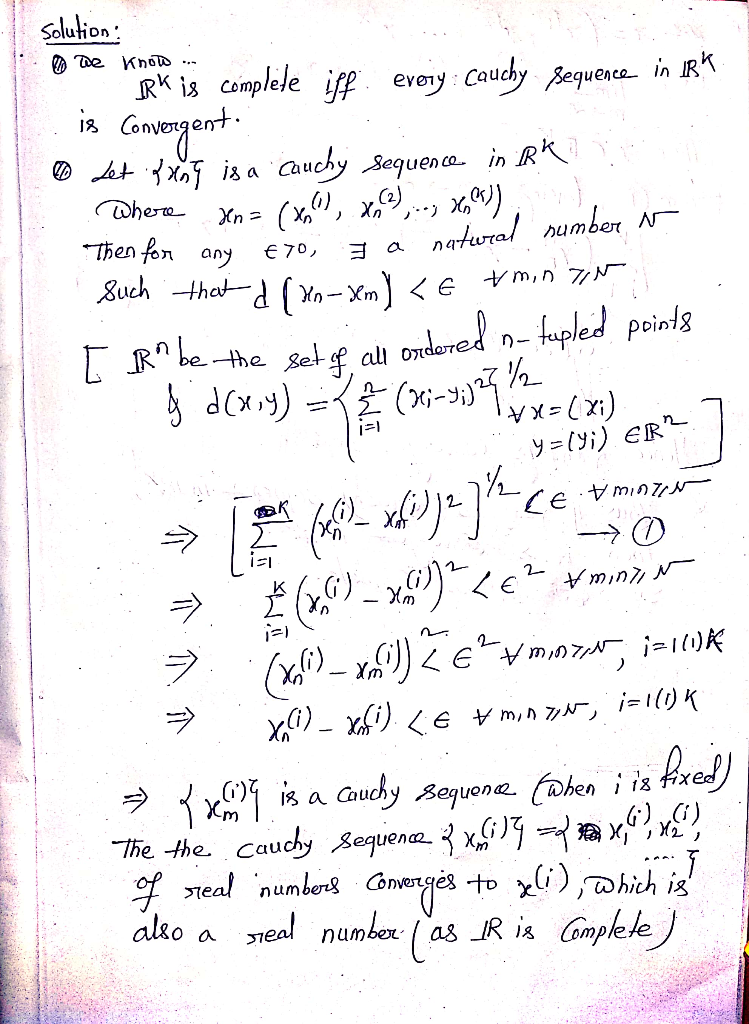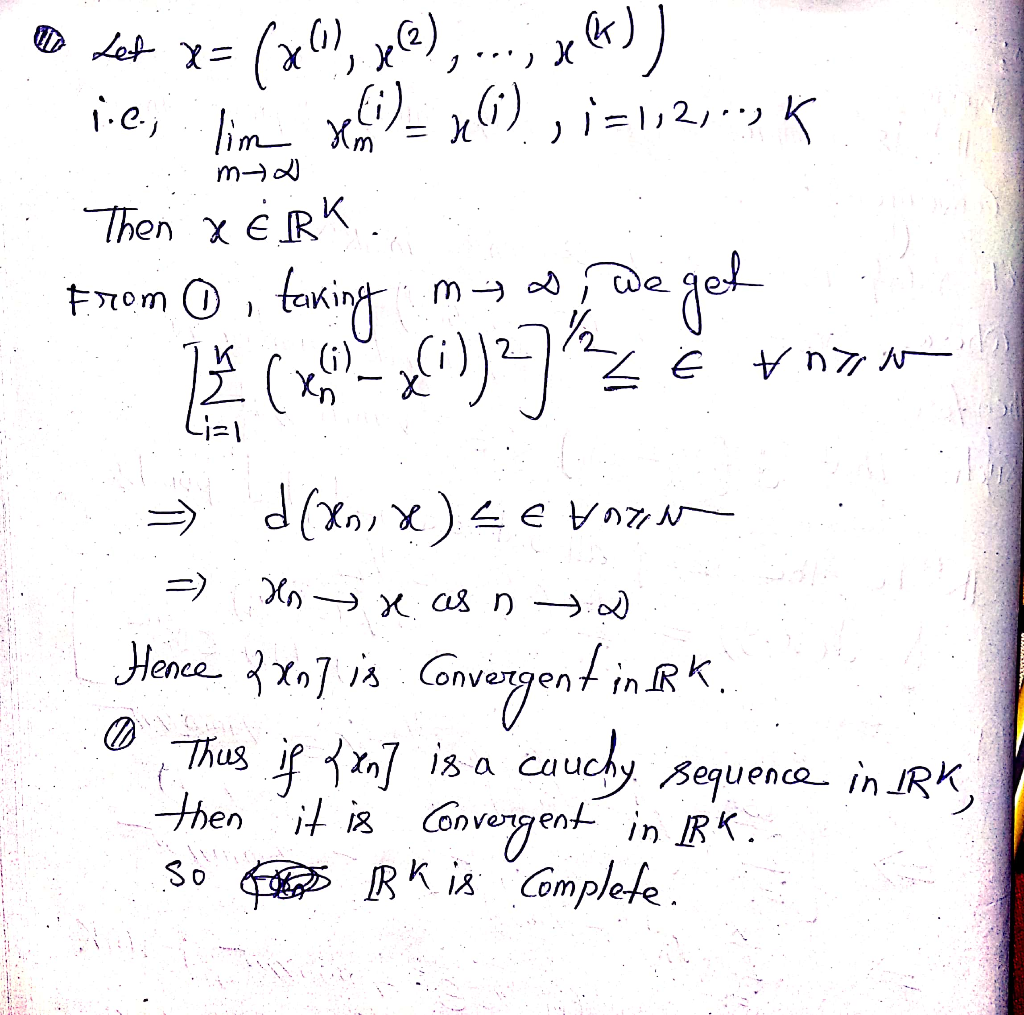# Let k ≥ 2. Use that R (the real numbers) is complete to show R^k is...

Let k ≥ 2. Use that R (the real numbers) is complete to show R^k is complete.

## Solutions

##### Expert Solution## Related Solutions

##### Let S = {2 k : k ∈ Z}. Let R be a relation defined on...
Let S = {2 k : k ∈ Z}. Let R be a relation defined on Q− {0} by x R y if x y ∈ S. Prove that R is an equivalence relation. Determine the equivalence class
##### 1. Let α < β be real numbers and N ∈ N. (a). Show that if...
1. Let α < β be real numbers and N ∈ N. (a). Show that if β − α > N then there are at least N distinct integers strictly between β and α. (b). Show that if β > α are real numbers then there is a rational number q ∈ Q such β > q > α. *********************************************************************************************** 2. Let x, y, z be real numbers.The absolute value of x is defined by |x|= x, if x ≥...
##### Let Rx denote the group of nonzero real numbers under multiplication and let R+ denote the...
Let Rx denote the group of nonzero real numbers under multiplication and let R+ denote the group of positive real numbers under multiplication. Let H be the subgroup {1, −1} of Rx. Prove that Rx ≈ R+ ⊕ H.
##### On the set S of all real numbers, define a relation R = {(a, b):a ≤ b}. Show that R is transitive.
On the set S of all real numbers, define a relation R = {(a, b):a ≤ b}. Show that R is transitive.
##### If R is the 2×2 matrices over the real, show that R has nontrivial left and...
If R is the 2×2 matrices over the real, show that R has nontrivial left and right ideals.? hello could you please solve this problem with the clear hands writing to read it please? Also the good explanation to understand the solution is by step by step please thank the subject is Modern Algebra
##### Let K = { s+t * 2^(1/2), such that s, t are Rational}. Show that K...
Let K = { s+t * 2^(1/2), such that s, t are Rational}. Show that K is a Field
##### Let R be the ring of all 2 x 2 real matrices. A. Assume that A...
Let R be the ring of all 2 x 2 real matrices. A. Assume that A is an element of R such that AB=BA for all B elements of R. Prove that A is a scalar multiple of the identity matrix. B. Prove that {0} and R are the only two ideals. Hint: Use the Matrices E11, E12, E21, E22.
##### 1) Show that if A is an open set in R and k ∈ R \...
1) Show that if A is an open set in R and k ∈ R \ {0}, then the set kA = {ka | a ∈ A} is open.
##### Let ? have the gamma (?,?) distribution. Show that ?(?≥2?(?))≤(2/?)^(r) [Remember that if ℎ is a...
Let ? have the gamma (?,?) distribution. Show that ?(?≥2?(?))≤(2/?)^(r) [Remember that if ℎ is a positive function of ?, then the value of ? that minimizes ℎhis the same as the value of ? that minimizes log(ℎ)]
##### 2. Define a relation R on pairs of real numbers as follows: (a, b)R(c, d) iff...
2. Define a relation R on pairs of real numbers as follows: (a, b)R(c, d) iff either a < c or both a = c and b ≤ d. Is R a partial order? Why or why not? If R is a partial order, draw a diagram of some of its elements. 3. Define a relation R on integers as follows: mRn iff m + n is even. Is R a partial order? Why or why not? If R is...